Definitions of Square Dance Calls and Concepts

Index -->  Plus  |  A1  |  A2  |  C1  |  C2  |  C3A  |  C3B  |  C4  |  NOL  |
Definitions (Text Only) -->  Plus  |  A1  |  A2  |  C1  |  C2  |  C3A  |  C3B  |  C4  |  NOL  |
 Find call:

 \$B8@8l(B view (admin)

2 x 4 \$B\$+\$i!%(B

Ends \$B\$O(B Split Circulate twice \$B\$r\$7(B, Centers \$B\$G30\$r8~\$\$\$F\$\$\$k?M\$O(B Crossover Circulate and Circulate \$B\$r\$7(B, Centers \$B\$GCf\$r8~\$\$\$F\$\$\$k?M\$O(B Circulate and Crossover Circulate \$B\$r\$7\$^\$9!%(B

2 x 4 \$B\$G=*\$o\$j\$^\$9!%(B

Drift Apart \$B\$O(B 2 \$B%Q!<%H\$N%3!<%k\$G\$9!%(B

Parallel Waves \$B\$+\$i(B: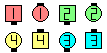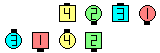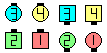Drift Apart\$B\$NA0(B \$BH>J,\$N\$H\$3\$m(B \$B8e(B
Columns \$B\$+\$i(B: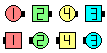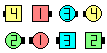Drift Apart\$B\$NA0(B \$B8e(B

\$BCmDrift Apart \$B\$O(B Tandem Cross Roll To A Line | Wave \$B\$HF1\$87k2L(B (Drift Apart \$B\$G\$OA4\$F\$N%@%s%5!<\$,(B Roll \$B\$G\$-\$k\$H\$\$\$&\$3\$H\$O\$J\$\$\$H\$\$\$&E@\$r=|\$-(B) \$B\$K\$J\$j\$^\$9!%(B

Crossover Circulate [A1] (Holman Hudspeth 1968): \$B0lHL2=\$5\$l\$?(B 2 x 4, \$B0lHL2=\$5\$l\$?(B Thar, \$B\$^\$?\$OB>\$NE,@Z\$J(B formation \$B\$+\$i!%(B1 \$B\$D\$NF0\$-\$H\$7\$F(B, Circulate & Half Sashay \$B\$r\$7\$^\$9!%(BCALLERLAB definition for Drift ApartChoreography for Drift ApartComments? Questions? Suggestions?

https://www.ceder.net/def/driftapart.php?language=japan&level=C3A
15-April-2021 07:25:30
Copyright © 2021 Vic Ceder.  All Rights Reserved.